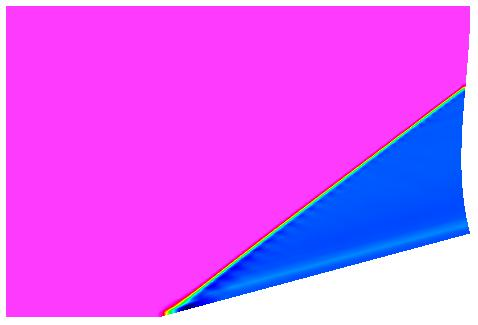NPARC Alliance Validation Archive
Validation Home   >   Archive   >   Oblique Shock on a 15 Degree Wedge at Mach 2.5

## Oblique Shock on a 15 Degree Wedge at Mach 2.5Figure 1. The shading of the Mach number for the Mach 2.5 flow
past a 15 degree wedge with the creation of an oblique shock.

### Flow Description

This verification case involves the computation of the supersonic flowfield past a wedge with a half-angle of 15 degrees. Fig. 1 shows the main features of the flow field. The freestream is a Mach number of 2.5. As the flow meets the leading edge of the wedge, an oblique shock is formed as the flow turns to become tangent with the wedge surface. The flow field past the shock is uniform.

Table 1. Freestream conditions.
Mach Pressure (psia) Temperature (R) Angle-of-Attack (deg) Angle-of-Sideslip (deg)
2.5 14.7 520.0 0.0 0.0

### Geometry

The leading edge of the wedge is located at coordinates (x=0,y=0) and is assumed to extend indefinately in the z coordinate. The half-angle of the wedge is 15 degrees measured from the x-axis. It is assumed to have and infinite length along the x-axis.

### Computational Domain and Boundary Conditions

The computational domain is bounded along the bottom by the symmetry plane of the wedge and the wedge surface. The inflow boundary is located at x = -0.5 ft. The outflow boundary is placed at x = 1.0 ft. The farfield boundary is placed at y = 1.0 ft to place it well above the oblique shock.

### Comparison Data

An analytic solution for this flow is well known [Anderson, 1982]. The solution gives the change in properties across the oblique shock as a function of the freestream Mach number and shock angle. The shock angle is known as an implicit function of the freestream Mach number and wedge half-angle (theta-beta-Mach relation). The inviscid flow conditions on each side of the oblique shock are uniform.

 Freestream Mach number 2.5 Wedge half-angle 15 degrees Angle of oblique shock 36.94490 degrees Mach number behind the oblique shock 1.873526 Pressure ratio (p2/p1) 2.467500 Density ratio (rho2/rho1) 1.866549 Temperature ratio (T2/T1) 1.321958

The Fortran program oblshk.f was used to compute these numbers. A tolerance of 1.0E-06 was specified for the "tol" input for oblshk.f.

### Computational Studies

Table 3. Computational studies.
Study Category Person Comments
wedge01 Example J.W. Slater 2D. Inviscid.
wedge02 Model J.W. Slater 2D. Inviscid. Grid sensitivity and order-of-accuracy study.
wedge03 Example J.W. Slater 3D. Laminar and turbulent flow.
wedge04 Check / Performance J.W. Slater Old (1997) comparison between NPARC2D and WIND 1.0.

### References

Anderson, J.D., Modern Compressible Flow , McGraw Hill Inc., New York, 1982.

Last Updated: Monday, 12-Mar-2012 14:12:15 EDT